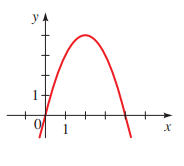# The x- and y- intercepts for the equation y = 4 x − x 2 and the graph of the equation is provided below,### Precalculus: Mathematics for Calcu...

6th Edition
Stewart + 5 others
Publisher: Cengage Learning
ISBN: 9780840068071### Precalculus: Mathematics for Calcu...

6th Edition
Stewart + 5 others
Publisher: Cengage Learning
ISBN: 9780840068071

#### Solutions

Chapter 1.8, Problem 53E
To determine

## To calculate: The x- and y- intercepts for the equation y=4x−x2 and the graph of the equation is provided below,Expert Solution

The x-intercepts are 0 and4 and y-intercept is 0.

### Explanation of Solution

Given information:

The equation y=4xx2 and the graph of the equation is provided below,Formula used:

The x-intercepts are the points on x-axis where the graph of the equation intersects the x-axis.

The y-intercepts are the points on y-axis where the graph of the equation intersects the y-axis.

Calculation:

Consider the provided equation y=4xx2 and the graph of the equation is sketched below,Observe from the graph that the curve intersects x-axis at two points 0 and 4 and y-axis only at one point that is 0.

Recall that the x-intercepts are the points on x-axis where the graph of the equation intersects the x-axis.

Substitute y=0 in the equation y=4xx2 ,

0=4xx2x(4x)=0

Either x=0 or 4x=0 .

Simplify it, either x=0 or x=4 .

Therefore, x-interceptsare 4 and 0.

Recall that the y-intercepts are the points on x-axis where the graph of the equation intersects the y-axis.

Substitute x=0 in the equation y=4xx2 ,

y=4(0)(0)2y=0

Therefore, y-intercept is 0.

Thus, the x-intercepts are0 and 4 and y-intercept is 0.

### Have a homework question?

Subscribe to bartleby learn! Ask subject matter experts 30 homework questions each month. Plus, you’ll have access to millions of step-by-step textbook answers!